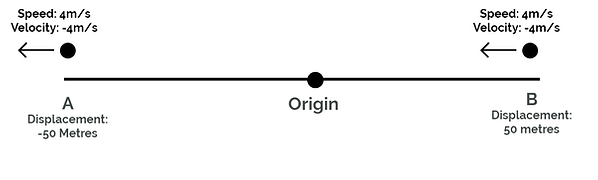top of page#### Motion and Derivatives

###### Displacement

Displacement is usually given by x or s. Displacement measures the position of an object from its origin. Displacement is similar to distance, with the exception it includes direction, positioning displacement as either positive or negative.

Consider the example below:Even though points A and B are of equal distance from the origin, point A has a displacement of negative -50 metres as it is to the left of the origin, hence a negative direction away from the origin. Point B has a positive displacement of 50 metres as it is to the right of the origin.

Anything to the left of the origin has a negative direction and hence negative displacement. Anything to the right of the origin has a positive direction and hence a positive displacement.

###### Velocity

Velocity is simply the speed of an object and the direction of that speed. If an object were to be moving towards to the left, it would have a negative velocity, while an objective moving towards the right would have a positive velocity.

Consider the below example. It is moving at a speed of 4m/s, however, as it is moving in a negative direction, it's velocity is -4m /s. It doesn't matter if it's displacement is positive or negative.

### Applications of DifferentiationThe below example shows a positive velocity of 4m/s as it the object is moving positively towards the right at 4m/s. It doesn't matter if it's displacement is positive or negative, at A and B, the velocity is still the same at 4m/s as the direction and speed is the same.

The velocity formula is always the differentiation of the displacement formula.

###### Acceleration

Acceleration is simple the rate of change in velocity. Consider the example below.As the velocity is increasing by 1m/s per second, the acceleration would be 1m/s^2.

If the velocity was decreasing by 1m/s each second, than the acceleration would be -1m/s^2.

The acceleration formula is the differentiation of the velocity formula or the double derivative of displacement.

###### In Summary...bottom of page